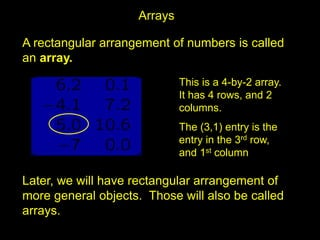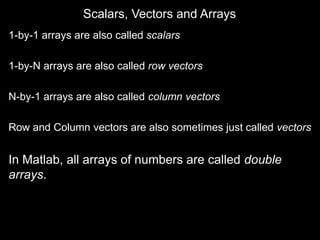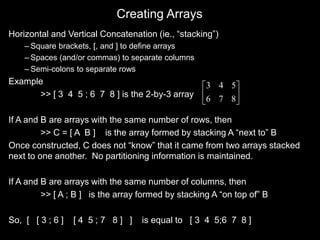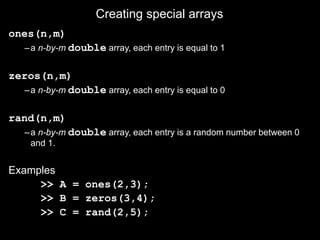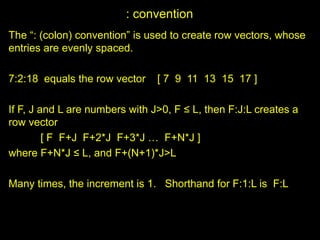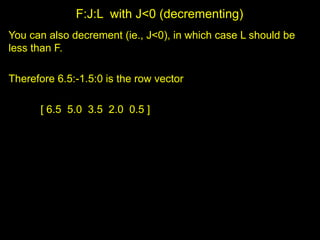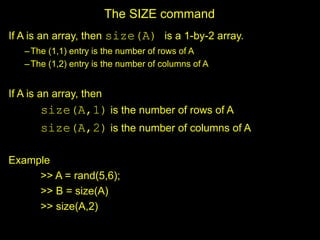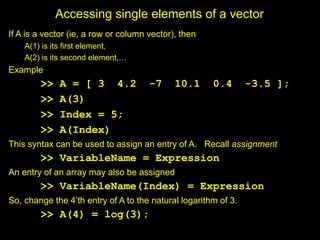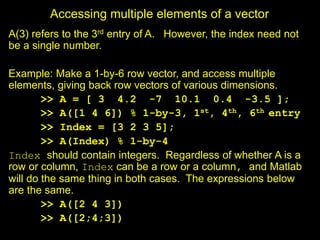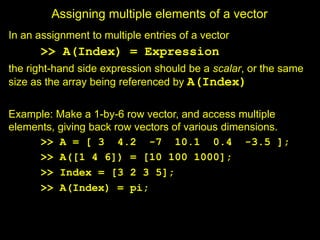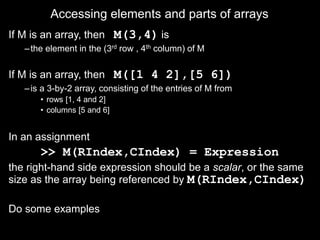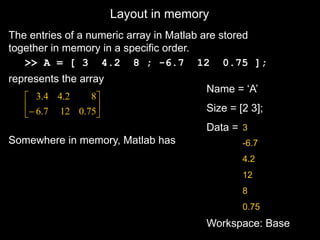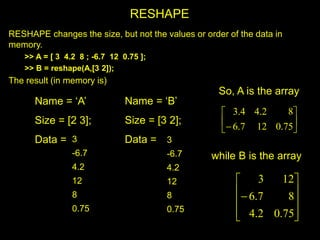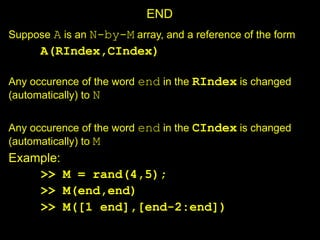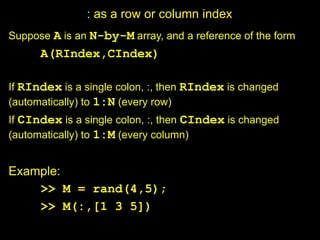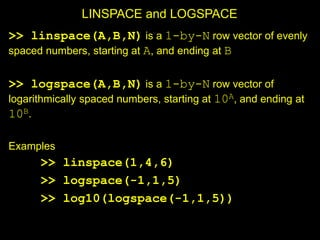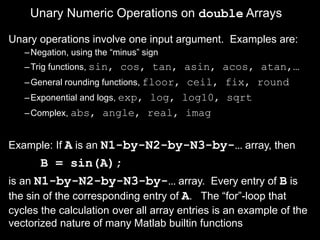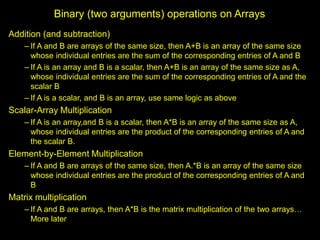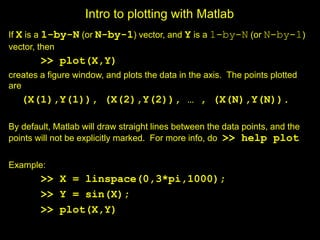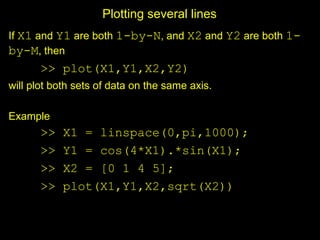1 of 21

### ArrayBasics.ppt

2. A rectangular arrangement of numbers is called an array. Later, we will have rectangular arrangement of more general objects. Those will also be called arrays. Arrays This is a 4-by-2 array. It has 4 rows, and 2 columns. The (3,1) entry is the entry in the 3rd row, and 1st column
3. Scalars, Vectors and Arrays 1-by-1 arrays are also called scalars 1-by-N arrays are also called row vectors N-by-1 arrays are also called column vectors Row and Column vectors are also sometimes just called vectors In Matlab, all arrays of numbers are called double arrays.
4. Creating Arrays Horizontal and Vertical Concatenation (ie., “stacking”) – Square brackets, [, and ] to define arrays – Spaces (and/or commas) to separate columns – Semi-colons to separate rows Example >> [ 3 4 5 ; 6 7 8 ] is the 2-by-3 array If A and B are arrays with the same number of rows, then >> C = [ A B ] is the array formed by stacking A “next to” B Once constructed, C does not “know” that it came from two arrays stacked next to one another. No partitioning information is maintained. If A and B are arrays with the same number of columns, then >> [ A ; B ] is the array formed by stacking A “on top of” B So, [ [ 3 ; 6 ] [ 4 5 ; 7 8 ] ] is equal to [ 3 4 5;6 7 8 ]       8 7 6 5 4 3
5. Creating special arrays ones(n,m) –a n-by-m double array, each entry is equal to 1 zeros(n,m) –a n-by-m double array, each entry is equal to 0 rand(n,m) –a n-by-m double array, each entry is a random number between 0 and 1. Examples >> A = ones(2,3); >> B = zeros(3,4); >> C = rand(2,5);
6. : convention The “: (colon) convention” is used to create row vectors, whose entries are evenly spaced. 7:2:18 equals the row vector [ 7 9 11 13 15 17 ] If F, J and L are numbers with J>0, F ≤ L, then F:J:L creates a row vector [ F F+J F+2*J F+3*J … F+N*J ] where F+N*J ≤ L, and F+(N+1)*J>L Many times, the increment is 1. Shorthand for F:1:L is F:L
7. F:J:L with J<0 (decrementing) You can also decrement (ie., J<0), in which case L should be less than F. Therefore 6.5:-1.5:0 is the row vector [ 6.5 5.0 3.5 2.0 0.5 ]
8. The SIZE command If A is an array, then size(A) is a 1-by-2 array. –The (1,1) entry is the number of rows of A –The (1,2) entry is the number of columns of A If A is an array, then size(A,1) is the number of rows of A size(A,2) is the number of columns of A Example >> A = rand(5,6); >> B = size(A) >> size(A,2)
9. Accessing single elements of a vector If A is a vector (ie, a row or column vector), then A(1) is its first element, A(2) is its second element,… Example >> A = [ 3 4.2 -7 10.1 0.4 -3.5 ]; >> A(3) >> Index = 5; >> A(Index) This syntax can be used to assign an entry of A. Recall assignment >> VariableName = Expression An entry of an array may also be assigned >> VariableName(Index) = Expression So, change the 4’th entry of A to the natural logarithm of 3. >> A(4) = log(3);
10. Accessing multiple elements of a vector A(3) refers to the 3rd entry of A. However, the index need not be a single number. Example: Make a 1-by-6 row vector, and access multiple elements, giving back row vectors of various dimensions. >> A = [ 3 4.2 -7 10.1 0.4 -3.5 ]; >> A([1 4 6]) % 1-by-3, 1st, 4th, 6th entry >> Index = [3 2 3 5]; >> A(Index) % 1-by-4 Index should contain integers. Regardless of whether A is a row or column, Index can be a row or a column, and Matlab will do the same thing in both cases. The expressions below are the same. >> A([2 4 3]) >> A([2;4;3])
11. Assigning multiple elements of a vector In an assignment to multiple entries of a vector >> A(Index) = Expression the right-hand side expression should be a scalar, or the same size as the array being referenced by A(Index) Example: Make a 1-by-6 row vector, and access multiple elements, giving back row vectors of various dimensions. >> A = [ 3 4.2 -7 10.1 0.4 -3.5 ]; >> A([1 4 6]) = [10 100 1000]; >> Index = [3 2 3 5]; >> A(Index) = pi;
12. Accessing elements and parts of arrays If M is an array, then M(3,4) is –the element in the (3rd row , 4th column) of M If M is an array, then M([1 4 2],[5 6]) –is a 3-by-2 array, consisting of the entries of M from • rows [1, 4 and 2] • columns [5 and 6] In an assignment >> M(RIndex,CIndex) = Expression the right-hand side expression should be a scalar, or the same size as the array being referenced by M(RIndex,CIndex) Do some examples
13. Name = ‘A’ Size = [2 3]; Data = Workspace: Base Layout in memory The entries of a numeric array in Matlab are stored together in memory in a specific order. >> A = [ 3 4.2 8 ; -6.7 12 0.75 ]; represents the array Somewhere in memory, Matlab has 3 -6.7 4.2 12 8 0.75        75 . 0 12 7 . 6 8 2 . 4 4 . 3
14. RESHAPE RESHAPE changes the size, but not the values or order of the data in memory. >> A = [ 3 4.2 8 ; -6.7 12 0.75 ]; >> B = reshape(A,[3 2]); The result (in memory is) 3 -6.7 4.2 12 8 0.75 Name = ‘A’ Size = [2 3]; Data = Name = ‘B’ Size = [3 2]; Data = 3 -6.7 4.2 12 8 0.75 while B is the array            75 . 0 2 . 4 8 7 . 6 12 3 So, A is the array        75 . 0 12 7 . 6 8 2 . 4 4 . 3
15. END Suppose A is an N-by-M array, and a reference of the form A(RIndex,CIndex) Any occurence of the word end in the RIndex is changed (automatically) to N Any occurence of the word end in the CIndex is changed (automatically) to M Example: >> M = rand(4,5); >> M(end,end) >> M([1 end],[end-2:end])
16. : as a row or column index Suppose A is an N-by-M array, and a reference of the form A(RIndex,CIndex) If RIndex is a single colon, :, then RIndex is changed (automatically) to 1:N (every row) If CIndex is a single colon, :, then CIndex is changed (automatically) to 1:M (every column) Example: >> M = rand(4,5); >> M(:,[1 3 5])
17. LINSPACE and LOGSPACE >> linspace(A,B,N) is a 1-by-N row vector of evenly spaced numbers, starting at A, and ending at B >> logspace(A,B,N) is a 1-by-N row vector of logarithmically spaced numbers, starting at 10A, and ending at 10B. Examples >> linspace(1,4,6) >> logspace(-1,1,5) >> log10(logspace(-1,1,5))
18. Unary Numeric Operations on double Arrays Unary operations involve one input argument. Examples are: –Negation, using the “minus” sign –Trig functions, sin, cos, tan, asin, acos, atan,… –General rounding functions, floor, ceil, fix, round –Exponential and logs, exp, log, log10, sqrt –Complex, abs, angle, real, imag Example: If A is an N1-by-N2-by-N3-by-… array, then B = sin(A); is an N1-by-N2-by-N3-by-… array. Every entry of B is the sin of the corresponding entry of A. The “for”-loop that cycles the calculation over all array entries is an example of the vectorized nature of many Matlab builtin functions
19. Binary (two arguments) operations on Arrays Addition (and subtraction) – If A and B are arrays of the same size, then A+B is an array of the same size whose individual entries are the sum of the corresponding entries of A and B – If A is an array and B is a scalar, then A+B is an array of the same size as A, whose individual entries are the sum of the corresponding entries of A and the scalar B – If A is a scalar, and B is an array, use same logic as above Scalar-Array Multiplication – If A is an array,and B is a scalar, then A*B is an array of the same size as A, whose individual entries are the product of the corresponding entries of A and the scalar B. Element-by-Element Multiplication – If A and B are arrays of the same size, then A.*B is an array of the same size whose individual entries are the product of the corresponding entries of A and B Matrix multiplication – If A and B are arrays, then A*B is the matrix multiplication of the two arrays… More later
20. Intro to plotting with Matlab If X is a 1-by-N (or N-by-1) vector, and Y is a 1-by-N (or N-by-1) vector, then >> plot(X,Y) creates a figure window, and plots the data in the axis. The points plotted are (X(1),Y(1)), (X(2),Y(2)), … , (X(N),Y(N)). By default, Matlab will draw straight lines between the data points, and the points will not be explicitly marked. For more info, do >> help plot Example: >> X = linspace(0,3*pi,1000); >> Y = sin(X); >> plot(X,Y)
21. Plotting several lines If X1 and Y1 are both 1-by-N, and X2 and Y2 are both 1- by-M, then >> plot(X1,Y1,X2,Y2) will plot both sets of data on the same axis. Example >> X1 = linspace(0,pi,1000); >> Y1 = cos(4*X1).*sin(X1); >> X2 = [0 1 4 5]; >> plot(X1,Y1,X2,sqrt(X2))